# 面试官:请算出走迷宫需要的最少步数

danielmou

0收藏

### 前言### 题解分析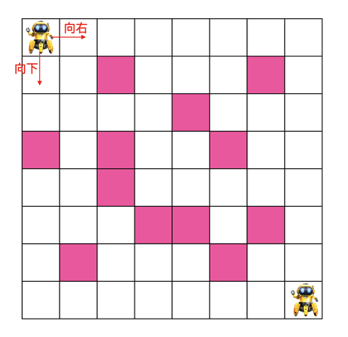``paths(start, end) = 1+min(paths(start下方格子， end), paths(start右边格子， end))``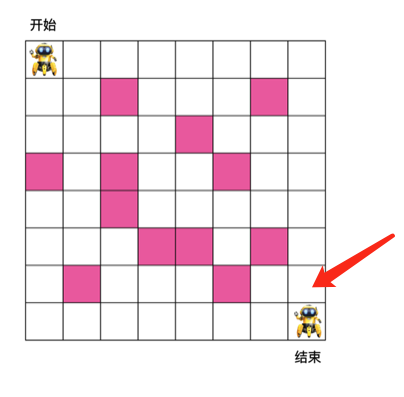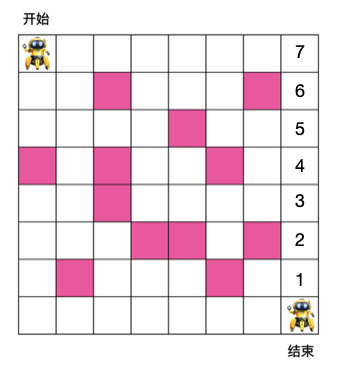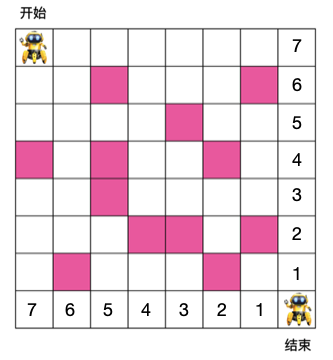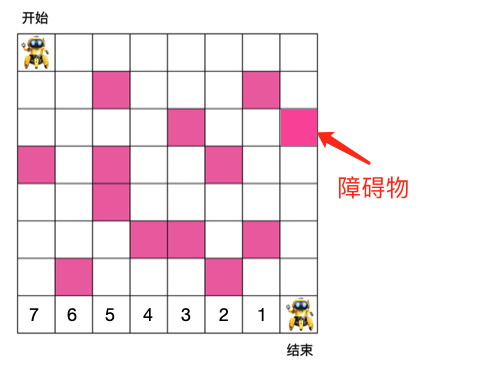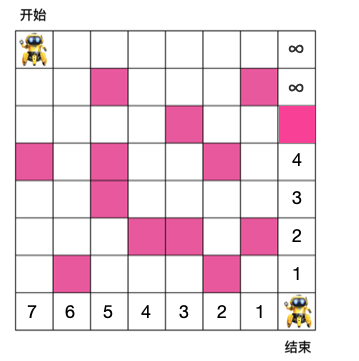``当前格子到出口的最小步数 = 1 + min(右格子到出口最小步数，下方格子到出口的最小步数)``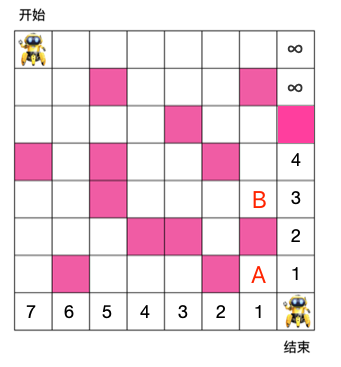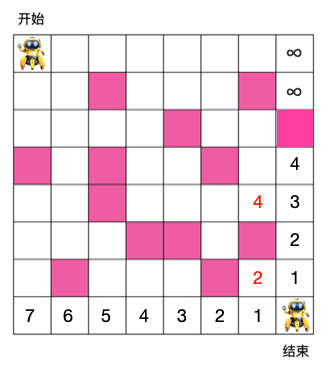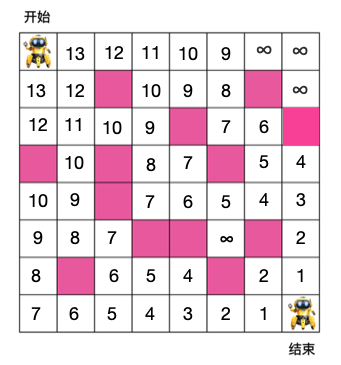``````public class Solution {

/**
* 初始化格子,假设为 8 x 8， -1 代表格子为障碍物
*/
private static final int GRIDS[][] = {
{0,0,0,0,0,0,0,0},
{0,0,-1,0,0,0,-1,0},
{0,0,0,0,-1,0,0,0},
{-1,0,-1,0,0,-1,0,0},
{0,0,-1,0,0,0,0,0},
{0,0,0,-1,-1,0,-1,0},
{0,-1,0,0,0,-1,0,0},
{0,0,0,0,0,0,0,0}
};

static private int getMinStep(){
/**
* 格子为 8 x 8
*/
int N = 8;

// 如果格子为障碍物，则此格子到出口的距离标记为无究大（这里用100000表示），代表此格子到出口不通
Integer infinity = 100000;

/**
* 初始化最右边的格子,从最后一列的倒数第二行开始（因为最后一个格子为出口）
*/
for (int row = N-2; row >= 0; row--) {
// 如果当前格子的下一个格子不是障碍物，则当前格子到出口的最小步数为 1 + 下个格子到出口的步数
if (GRIDS[row+1][N-1] != -1) {
GRIDS[row][N-1] = 1 + GRIDS[row+1][N-1];
} else {
// 如果下一个格子是障碍物，则此格子到出口的步数设置为无穷大（代表此路不通），这里用正整数的最大值表示
GRIDS[row][N-1] = infinity;
}
}

/**
* 初始化最底部的格子,从最后一行的倒数第二列开始（因为最后一个格子为出口）
*/
for (int column = N-2; column >= 0; column--) {
// 如果当前格子右边的格子不是障碍物，则当前格子到出口的最小步数为 1 + 右格子到出口的步数
if (GRIDS[N-1][column+1] != -1) {
GRIDS[N-1][column] = 1 + GRIDS[N-1][column+1];
} else {
// 如果是障碍物，则到出口的步数为无穷大，这里用正整数的最大值表示
GRIDS[N-1][column] = infinity;
}
}

/**
* 从右到左，从下到上填满每个格子的值，由于最右和最底部的格子都初始化了，
* 所以从倒数第二行，倒数第二列开始遍历
*/
for (int i = N - 2; i >= 0; i--) {
for (int j = N - 2; j >= 0; j--) {
// 说明是障碍物，所在格子到出口步数显然为无穷大（代表此路不通）
if (GRIDS[i][j] == -1) {
GRIDS[i][j] = infinity;
continue;
}
/**
* 当前格子到出口的最小步数为1+(右边的格子到出口的步数与下格子到出口步数之和的最小值)
*/
GRIDS[i][j] = 1 + Math.min(GRIDS[i+1][j], GRIDS[i][j+1]);
}
}

/**
* 遍历完之后起点格子对应的值即为最终所求的解
*/
return GRIDS;
}

public static void main(String[] args){
int result = Solution.getMinStep();
System.out.println("result = " + result);
}
}````````当前格子到出口的最小步数 = 1 + min(右格子到出口最小步数，下方格子到出口的最小步数)``